# Odd positive power of sine function

Jump to: navigation, search

## Definition

This page is about functions of the form:$x \mapsto (\sin x)^n$

where$n$ is an odd positive integer, i.e.,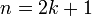$n = 2k + 1$ for$k$ a nonnegative integer.

In other words, the function is the composite of an odd positive power function and the sine function.

## Integration

### First antiderivative: as a polynomial in cosine

We consider$n = 2k + 1$,$k$ a nonnegative integer: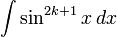$\int \sin^{2k + 1} x \, dx$

Rewrite$\sin^{2k + 1}x = \sin^{2k}x \sin x = (1 - \cos^2x)^k \sin x$. We get: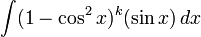$\int (1 - \cos^2x)^k(\sin x) \, dx$

Set$u = \cos x$, and we get:$\int -(1 - u^2)^k \, du$

This is a polynomial integration in$u$. After obtaining the answer, we plug back$u = \cos x$.

Here is the general integration in terms of binomial coefficients: [SHOW MORE]

Note that in all instances, the answer is an odd polynomial of the cosine function.

We consider the integration in some small cases:$k$$n = 2k + 1$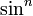$\sin^n$ Antiderivative as a polynomial in$\cos$
0 1 sine function$\! -\cos x + C$
1 3 sine-cubed function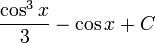$\frac{\cos^3x}{3} - \cos x + C$
2 5 fifth power of sine function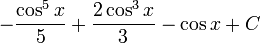$- \frac{\cos^5x}{5} + \frac{2\cos^3x}{3} - \cos x + C$
3 7 seventh power of sine function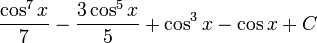$\frac{\cos^7x}{7} - \frac{3 \cos^5x}{5} + \cos^3x - \cos x + C$